# HBSE 9th Class Maths Notes Chapter 6 Lines and Angles

Haryana State Board HBSE 9th Class Maths Notes Chapter 6 Lines and Angles Notes.

## Haryana Board 9th Class Maths Notes Chapter 6 Lines and Angles

Introduction
In previous chapter, we have already studied axioms, postulate and some terms allied to geometry. In this chapter, we shall study the angles and their properties and also the properties of the angles formed when a line intersects two or more parallel lines at distinct points.

An architect cannot draw the plan of the multistoried building without the knowledge of the properties of parallel lines, intersecting lines and angles.

In science, to study the refraction property of light when a ray enters from one medium to other medium, we use the properties of parallel lines and intersecting lines. We have to represent forces by direct line segments to study the net effect of the forces on the body.

Key Words
→ Architect: One who designs building and supervises their construction.

→ Congruent: Having the same size and shape.

→ Intersect: Two geometrical figures or curves are said to intersect if they have at least one point in common.

→ Converse: The converse of an implication p ⇒ q is the implication q ⇒ p. If an implication is true, then its converse may or may not be true.

→ Concurrent: A number of lines are said to be concurrent, if there is a point through which they all pass.

→ Incident Ray: The incident ray is the ray of light that strikes the surface before reflection, transmission or absorption.

→ Reflected Ray: A ray extending outward from a point.

→ Angle of Incidence: The angle between the direction of an approaching emission and the normal to the surface upon which it is incident.

→ Angle of Reflection: The angle between the direction of propagation of a reflected emission and the normal to the reflecting surface.1. Basic Terms and Definitions : (a) Angle: An angle is formed when two rays or two line segments have a common end point. The two rays which form an angle are called the arms and the point at which they meet is called the vertex of the angle.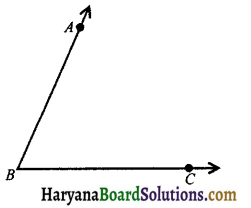The adjacent figure represent an angle ABC or ∠ABC or ∠CBA or simply B. AB and BC are the arms of the angle and their common point B is the vertex.

∠ABC divides the plane containing it into two parts:
(i) Interior of an angle
(ii) Exterior of an angle.
(i) Interior of an angle: The interior of an angle ABC is the set of all points in its plane, which lie on the same side of BC as A and also on the same side of AB as C. In the adjoining figure, P is a point in the interior of an angle ABC.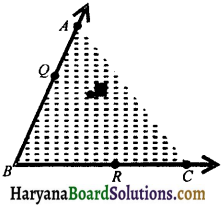(ii) Exterior of an angle : The exterior of an ∠ABC is the set of all points in its plane, which do not lie on the angle or in its interior.
In the adjoining figure, the point Q is exterior of the ∠ABC.
Note: Point R lies on the angle ∠ABC.

(b) Measure of an angle: The amount of turning of a ray from the initial position of BC to BA is called the measure of ∠ABC which is written as m∠ABC. The unit of angle measure is a standard angle, called a degree.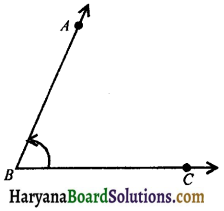If the measure of an angle ABC is x degree, then we write
m∠ABC = x°.
Note: 1° = 60′ (i.e., 60 minutes),
1′ = 60″ (60 seconds)

(c) Congruent or equal angles: If the measures of the angles are same then they said to be equal or congruent.
For examples:
If m∠ABC = m∠PQR,
then ∠ABC ≅ ∠PQR
or if ∠ABC ≅ ∠DEF,
then m∠ABC = m∠DEF.

(d) Types of angles: (i) Right angle: The angle which measures equal to 90°, is called a right angle. In the figure 6.4, ∠PQR is a right angle.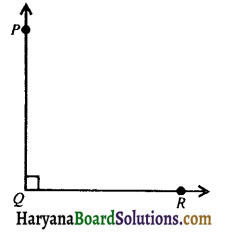(ii) Acute angle: The angle which measures less than one right angle (i.e., less than 90°) is called an acute angle.
In the figure 6.5, ∠PQR is an acute angle.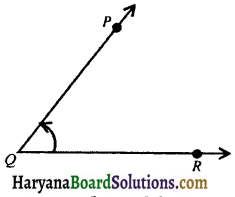(iii) Obtuse angle: The angle which measures more than 90° and less than 180° is called an obtuse angle. In the figure 6.6, ∠PQR is an obtuse angle.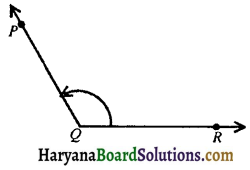(iv) Straight angle: The angle which measures equal to two right angles (i.e., equal to 180°) is called a straight angle.
In the figure 6.7, ∠PQR is a straight angle.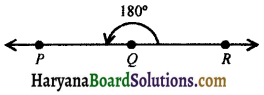(v) Reflex angle: The angle which measures more than 180° and less than 360° is called a reflex angle.
In the figure 6.8, ∠PQR is a reflex angle.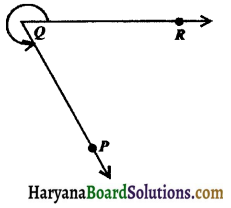(vi) Complete angle: The angle which measures is 4 right angles (i.e., 360°) is called a complete angle.
In the figure 6.9, ∠QPR is a complete angle.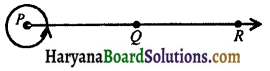(vii) Complementary angles: If the sum of two angles is 90°, they are called complementary angles eg., if ∠a + ∠b= 90°, then ∠PQS and ∠RQS are complementary angles.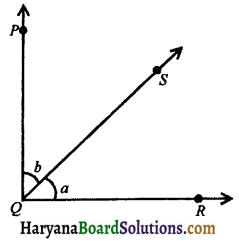(viii)Supplementary angles: If the sum of two angles is 180°, they are called. supplimentary angles eg., if ∠x + ∠y = 180°, then ∠PQS and ∠RQS are supplementary angles.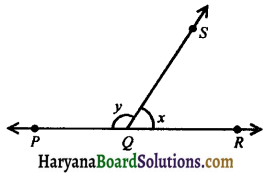(e) Bisetor of an angle: A ray is called the bisector of an angle if it divides the angle into two equal parts.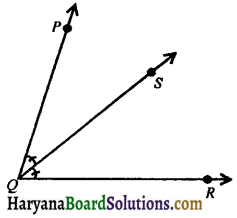In the figure 6.12, ray QS is the bisector of ∠PQR, then
∠PQS = ∠RQS.2. Pairs of Angles: (i) Adjacent angles: Two angles are called adjacent angles, if:
(i) they have the same vertex.
(ii) they have a common arm.
(iii) other arms of these angles are on opposite sides of the common arm.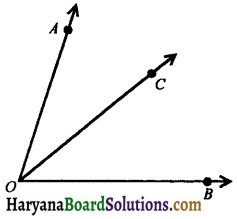In the figure 6.13, ∠AOC and ∠BOC have the common vertex O and have a common arm OC and other arms OA and OB lie on the opposite sides of the common arm OC. Therefore, ∠AOC and ∠BOC are adjacent angles.

(ii) Linear pair angles: Two adjacent angles are said to form a linear pair of angles, if their non-common arms are two opposite rays.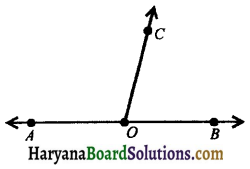In the figure 6.14, ∠AOC and ∠BOC are two adjacent angles and OA and OB are two opposite rays. Therefore, ∠AOC and ∠BOC form a linear pair of angles.

Axiom 6.1: If a ray stands on a line, then the sum of two adjacent angles so formed is 180°.
Recall that when the sum of two adjacent angle is 180°, then they are called a linear pair of angles.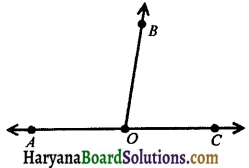In the figure 6.15, ray OB stands on the line AC. It forms adjacent angles ∠AOB and ∠COB.
∵ ∠AOB + ∠COB = 180° and OA and OC are opposite rays. Thus, two angles form a linear pair of angles. The above axiom can be stated in the reverse ways as below:
Axiom 6.2: If the sum of two adjacent angles is 180°, then the non-common arms of the angles form a line.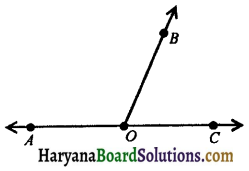In the figure 6.16, ∠AOB and ∠COB are adjacent angles, such that
∠AOB + ∠COB = 180°
⇒ ∠AOC = 180°,
[∵ ∠AOC = ∠AOB + ∠COB]
⇒ ∠AOC is a straight line.
Hence, AOC is a straight line.
The two axioms above together is called the linear pair axiom.

(iii) Vertically opposite angles: Two angles are called a pair of vertically opposite angles, if their arms form two pairs of opposite rays.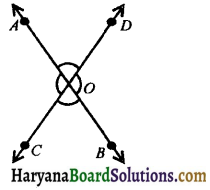In the figure 6.17, two lines AB and CD intersect at O, then two pairs of vertically opposite angles are formed.
(i) ∠AOC and ∠BOD
(ii) ∠AOD and ∠BOC.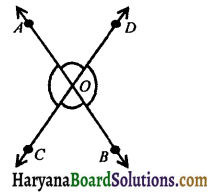Theorem 6.18.
If two lines intersect each other, then the vertically opposite angles are equal.
Given: Two lines AB and CD intersect at a point O.
To prove : (i) ∠AOC = ∠BOD
(ii) ∠AOD = ∠BOC.
Proof. Since ray OA stands on line CD.
∴∠AOC + ∠AOD = 180°,
(Linear pair axiom) …….(i)
Ray OD stands on line AB.
∴ ∠AOD + ∠BOD = 180°,
(Linear pair axiom) …….(ii)
From (i) and (ii), we have
∠AOC + ∠AOD = ∠AOD + ∠BOD
⇒ ∠AOC = ∠BOD
Similarly, we can prove that
∠AOD = ∠BOC. Hence provedParallel Lines and a Transversal: A line which intersects two or more given lines at distinct points is called a transversal of the given lines.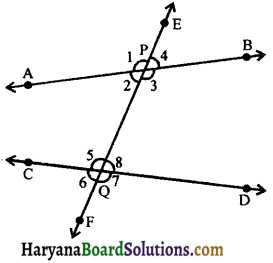In the figure 6.32, AB and CD are two lines and a transversal EF intersects them at P and Q respectively. The three lines determine eight angles, four angles at P namely ∠1, ∠2, ∠3, ∠4 and remaining four angles at Q namely ∠5, ∠6, ∠7 and ∠8.

In the figure, ∠2, ∠3, ∠5 and ∠8 are called interior angles and ∠1, ∠4, ∠6 and ∠7 are called exterior angles. We classify these eight angles in the following groups:
(a) Corresponding angles: (i) ∠1 and ∠5, (ii) ∠4 and ∠8, (iii) ∠2 and ∠6, (iv) ∠3 and ∠7.
(b) Alternate interior angles: (i) ∠2 and ∠8, (ii) ∠3 and ∠5.
(c) Alternate Exterior angles: (i) ∠1 and ∠7, (ii) ∠4 and ∠6.
(d) Interior angles on the same side of the transversal: (i) ∠2 and ∠5, (ii) ∠3 and ∠8.
Interior angles on the same side of the transversal are also referred to as consecutive interior angles or allied angles or co-interior angles.

Some Important Relations
If AB || CD and transversal XY cuts them (see in the figure 6.33) then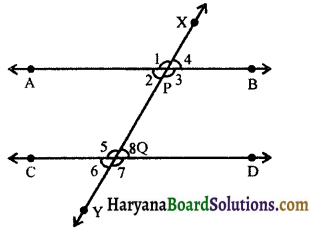(i) Corresponding angles are equal i.e., ∠1 = ∠5, ∠4 = ∠8, ∠2 = ∠6 and ∠3 = ∠7.
(ii) Alternate interior angles are equal i.e., ∠2 = ∠8 and ∠3 = ∠5.
(iii) Alternate exterior angles are equal i.e., ∠1 = ∠7 and ∠4 = ∠6.
(iv) Co-interior angles are supplementary i.e., ∠2 + ∠5 = 180° and ∠3 + ∠8 = 180°.
From this we conclude the following axioms:
Axiom 6.3: If a transversal intersects two parallel lines, then each pair of corresponding angles are equal. It is called corresponding angles axiom also.
Axiom 6.4: If a transversal intersects two lines such that a pair of corresponding angles is equal, then the two lines are parallel to each other. It is called converse of corresponding angles axiom.

Theorem 6.2:
If a transversal intersects two parallel lines, then each pair of alternate interior angles is equal.
Given: A transversal line XY cuts the parallel lines AB and CD at P and Q respectively.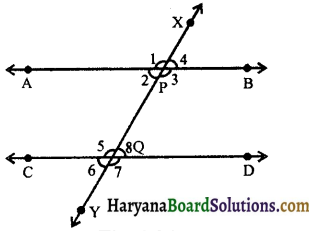To prove : ∠2 = ∠8 and ∠3 = ∠5.
Proof :
∠4 = ∠2 ……(i)
(vertically opposite angles)
∠4 = ∠8 ……(ii)
(corresponding angles axiom)
From (i) and (ii), we get
∠2 = ∠8
Again, ∠1 = ∠3 ……(iii)
(vertically opposite angles)
∠1 = ∠5 ……(iv)
(corresponding angles axiom)
From (iii) and (iv), we get
∠3 = ∠5
Hence, ∠2 = ∠8 and ∠3 = ∠5.
ProvedTheorem 6.3:
(Converse of theorem 6.2) : If a transversal intersects two lines such that a pair of alternate interior angles is equal, then the two lines are parallel.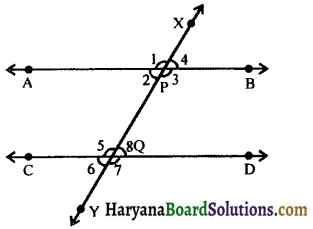Given: A transversal line XY intersects two lines AB and CD at P and Q respectively such that ∠2 and ∠8 are a pair of alternate interior angles and ∠2 = ∠8.
To prove : AB || CD.
Proof :
∠2 = ∠8, (given) ……(i)
∠2 = ∠4 …….(ii)
(vertically opposite angles)
From (i) and (ii), we get
∠4 = ∠8
Thus, a pair of corresponding angles 24 and 28 are equal. Therefore, by converse of corresponding angle axiom, we have
AB || CD.
Hence proved

Theorem 6.4:
If a transversal intersects two parallel lines, then each pair of interior angles on the same side of the transversal is supplementary.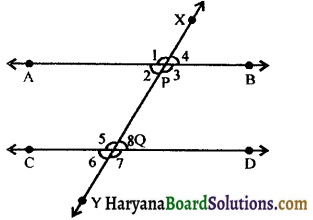Given: A transversal line XY intersects two parallel lines AB at P and CD at Q making two pairs of consecutive interior angles ∠2, ∠5, ∠3 and ∠8.
To prove :
∠2 + ∠5 = 180° and ∠3 + ∠8 = 180°.
Proof: Ray PB stands on line XY.
∴ ∠3 + ∠4 = 180
(Linear pair axiom)
But ∠8 = ∠4
(corresponding angles axiom)
∴ ∠3 + ∠8 = 180° ……(i)
Now, ray QC stands on line XY.
∴ ∠5 + ∠6 = 180°,
(Linear pair axiom)
But ∠6 = ∠2,
(corresponding angles axiom)
∴ ∠5 + ∠2 = 180°
⇒ ∠2 + ∠5 = 180° ……(ii)
Hence, ∠2 + ∠5 = 180°
and ∠3 + ∠8 = 180°.
Proved.Theorem 6.5.
(Converse of theorem 6.4): If a transversal intersects two lines such that a pair of interior angles on the same side of the transversal is supplementary, then the two lines are parallel.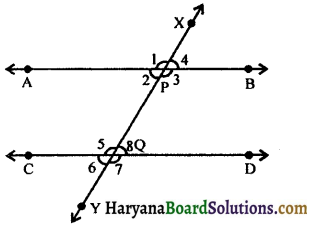Given: A transversal line XY intersects two lines AB at P and CD at Q such that ∠2 and ∠5 are pair of consecutive interior angles and ∠2 + ∠5 = 180°.
To prove : AB || CD.
Proof: Since, Ray PA stands on line XY.
∴ ∠1 + ∠2 = 180°
(Linear pair axiom) …..(i)
∠2 + ∠5 = 180° (Given) …..(ii)
From (i) and (ii), we get
∠1 + ∠2 = ∠2 + ∠5
⇒ ∠1 = ∠5
Thus, a pair of corresponding angles ∠1 and ∠5 are equal.
Therefore, by converse of corresponding angles axiom, we have
AB || CD. Hence proved.Lines Parallel to Same Line:
Theorem 6.6:
Lines which are parallel to the same line are parallel to each other.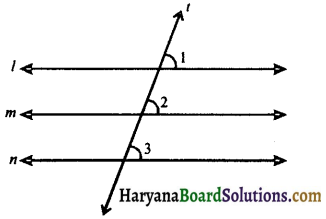Given: l || m and n || m.
To prove : l || m
Construction: Draw a transversal lines t for the lines l, m and n.
Proof: Since, l || m (Given)
∠1 = ∠2 …….(i)
(Corresponding angles axiom)
Since, n || m (Given)
∠3 = ∠2 …….(ii)
(Corresponding angles axiom)
From (i) and (ii), we get
∠1 = ∠3
But these are corresponding angles.
⇒ l || n,
[By converse of corresponding angles]
Hence proved1. Triangle: A plane figure bounded by three straight lines, is called a triangle. It is usually denoted by the Greek letter Δ (delta).
The figure, given along side, shows a triangle ABC (ΔABC) bounded by three sides AB, BC and CA. Triangle ABC has three vertices, namely A, B and C and it has three angles, namely ∠A, ∠B and ∠C.
Note: If side BC of ΔABC is produced upto any point D, the angle ∠ACD so formed, is called exterior angle at C, where as the angles A and B are called its interior opposite angles.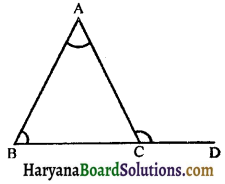2. Kinds of Triangles: (a) With regard to their sides:
(i) Equilateral triangle: If all the sides of a triangle are equal, it is called an equilateral triangle.
In the given figure, ΔABC is an equilateral triangle because AB = BC = CA. Also, all the angles of an equilateral triangle are equal to each other and so each angle = 60°.

(ii) Isosceles triangle: If at least two sides of a triangle are equal, it is called an isosceles triangle. In the given figure, ΔABC is an isosceles triangle, because AB = AC.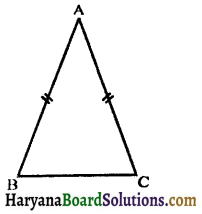In an isosceles triangle, two angles of opposite equal side are also equal.

(iii) Scalene triangle: If all the sides of a triangle are unequal, it is called an scalene triangle.
The figure below shows a scalene triangle because AB ≠ BC ≠ AC.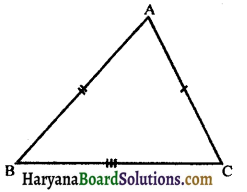In a scalene triangle, all its angles are also unequal.

(b) With regard to their angles: (i) Acute angled triangle: When each angle of a triangle is acute (i.e., less than 90°), it is called an acute angled triangle.
The given figure 6.60, shows an acute angled triangle, because each angle of the triangle is less than 90°.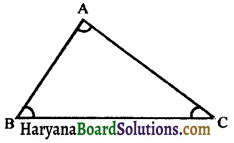(ii) Right angled triangle: When one angle of a triangle is right angle (ie., equal to 90°), it is called right angled triangle, the side opposite to the right angle is called its hypotenuse and the remaining two sides are called its legs.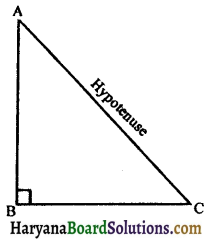The given figure 6.61, shows a right angled triangle because ∠B = 90°. In triangle ABC, AC is hypotenuse and AB and BC are its legs. Each of the other two angles of the right triangle is acute.

(iii) Obtuse angled triangle: When one angle of a triangle is obtuse (i.e., greater than 90° but less than 180°), it is called an obtuse angled triangle. The given figure 6-62, shows an obtuse angled triangle, because B is obtuse.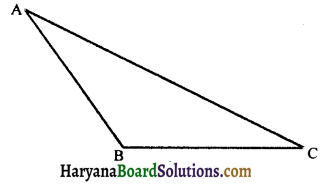In an obtuse angled triangle, each of the other two angles is acute.

3. Some Important Terms Related to the Traingle: (i) Median : The median of a triangle, corresponding to any side, is the line joining the mid point of that side with the opposite vertex. e.g.,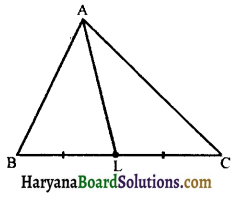AL is the median corresponding to side BC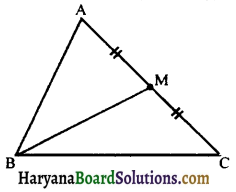CN is the median corresponding to side AB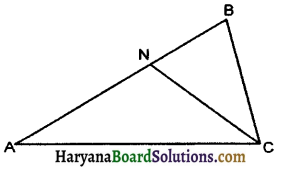A triangle has three medians and all three medians are always concurrent i.e., they intersect each other at one point only.

(ii) Centroid: The point of intersection of all the three medians of a triangle is called its centroid. In the given tigure 6-66, three medians AD, BE and CF intersect at the point G. Therefore, G is the centroid of the triangle ABC.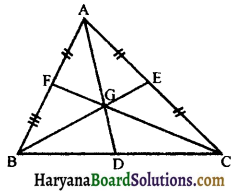(iii) Altitude: An altitude of a triangle, corresponding to any side, is the length of perpendicular drawn from the opposite vertex to that side. e.g.,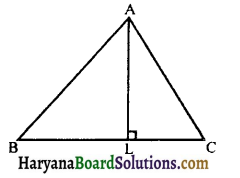AL is the altitude corresponding to side BC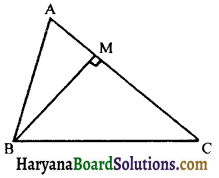BM is the altitude corresponding to side AC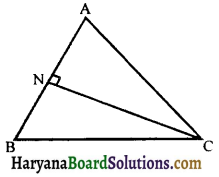CN is the altitude co-rresponding to side AB A triangle has three altitudes and all three altitudes are always concurrent i.e., they intersect each other at one point only.

(iv) Ortho centre: The point of intersection of all the three altitudes of a triangle is called ortho centre.
In the given figure 6.69, O is the ortho centre of triangle ΔABC.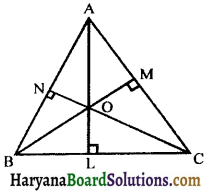4. Angle sum property of a triangle: In previous classes, we have studied that sum of the angles of a triangle is 180°. We can prove this statement using the theorem related to parallel lines.Theorem 6.7:
The sum of the angles of a triangle is 180°.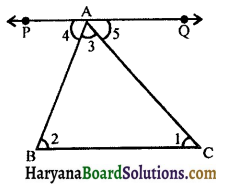Given: A triangle ΔABC and ∠1 + ∠2 + ∠3 are its angles
To prove : ∠1 + ∠2 + ∠3 = 180°.
Construction: Through A, draw a line PQ parallel to BC.
Proof: PQ || BC and AB is the transversal.
∠2 = ∠4 ……(i)
(Alternate interior angles)
Again, PQ || BC and AC is the transversal.
∴ ∠1 = ∠5, ……(ii)
(Alternate interior angles)
Adding (i) and (ii), we get
∠2 + ∠1 = ∠4 + ∠5
⇒ ∠1 + ∠2 + ∠3 = ∠4 + ∠3 + ∠5,.
But, ∠4 + ∠3 + ∠5 = 180°,
(Linear pair axiom)
∴ ∠1 + ∠2 + ∠3 = 180°
Hence, the sum of three angles of a triangle is 180°.
Hence Proved

Theorem 6.8:
If a side of a triangle is produced, then the exterior angle so formed is equal to the sum of the two interior opposite angles.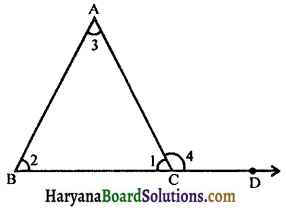Given: A ΔABC whose side BC has been produced to D such that ∠4 is its exterior angle.
To prove : ∠4 = ∠2 + ∠3.
Proof: We know that,
Sum of the angles of a triangle is 180°,
(By theorem 6.7)
⇒ ∠1 + ∠2 + ∠3 = 180°…(i)
∠1 + ∠4 = 180°…(ii)
(Linear pair axiom)
From (i) and (ii), we get
∠1 + ∠4 = ∠1 + ∠2 + ∠3
⇒ ∠4 = ∠2 + ∠3. Hence proved

Corollary: An exterior angle of a triangle is greater than either of its interior opposite angles.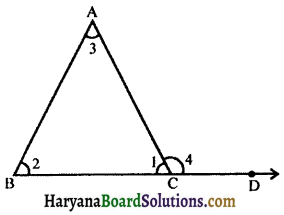Proof: We know that an exterior angle of a triangle is equal to the sum of the interior opposite angles (By theorem 6.8)
∴ ∠4 = ∠2 + ∠3
∴ ∠4 > ∠2 and ∠4 > ∠3
Hence, an exterior angle of a triangle is greater than each interior opposite angle.